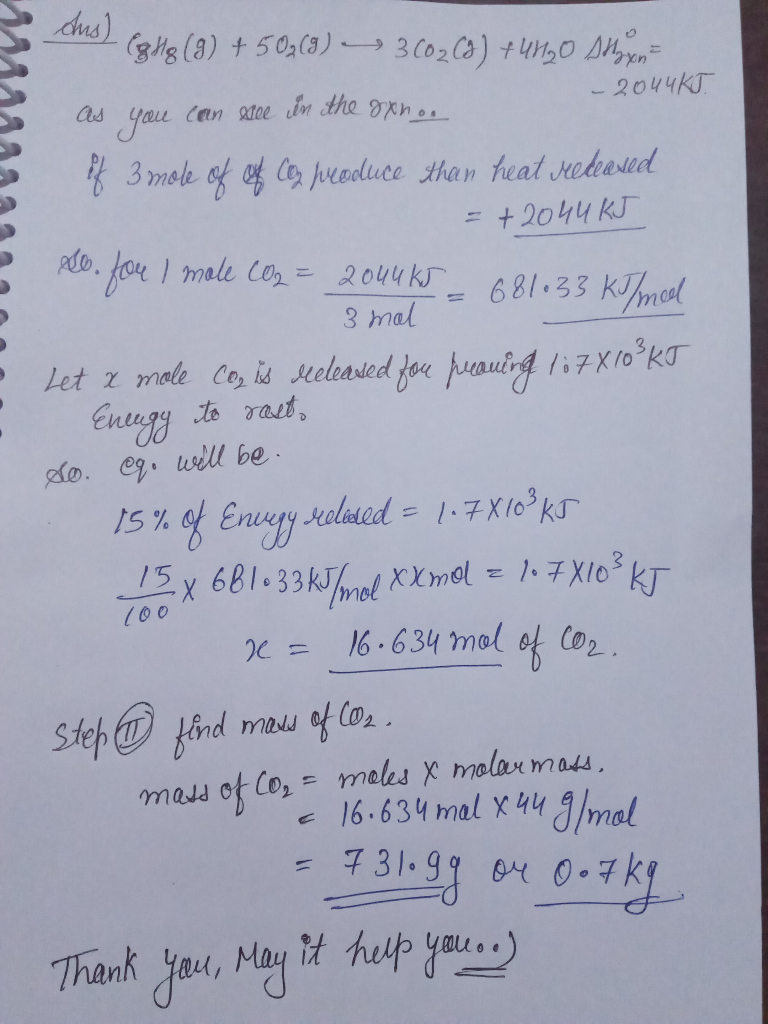Question

# The propane fuel (C3H8) used in gas barbeques burns according to this thermochemical equation. C3H8(g)+5O2(g)→3CO2(g)+4H2O(g)ΔH∘rxn=−2044kJ If...

The propane fuel (C3H8) used in gas barbeques burns according to this thermochemical equation.
C3H8(g)+5O2(g)→3CO2(g)+4H2O(g)ΔH∘rxn=−2044kJ

If a pork roast must absorb 1.7×103 kJ to fully cook, and if only 15 % of the heat produced by the barbeque is actually absorbed by the roast, what mass of CO2 is emitted into the atmosphere during the grilling of the pork roast?#### Earn Coins

Coins can be redeemed for fabulous gifts.

Similar Homework Help Questions
• ### The propane fuel (C3H8) used in gas barbeques burns according to the following thermochemical equation: C3H8(g)+5O2(g)...

The propane fuel (C3H8) used in gas barbeques burns according to the following thermochemical equation:C3H8(g)+5O2(g)→3CO2(g)+4H2O(g) ΔH rxn= 2217kJPart AIf a pork roast must absorb 1500kJ to fully cook, and if only 12% of the heat produced by the barbeque is actually absorbed by the roast, what mass of CO2 is emitted into the atmosphere during the grilling of the pork roast?Express your answer using two significant figures. m=_____________________g

• ### The propane fuel (C3H8) used in gas barbecues burns according to the following equation: C3H8(g)+5O2(g)→3CO2(g)+4H2O(g)ΔH∘rxn=−2044kJ PART...

The propane fuel (C3H8) used in gas barbecues burns according to the following equation: C3H8(g)+5O2(g)→3CO2(g)+4H2O(g)ΔH∘rxn=−2044kJ PART A: If a pork roast must absorb 2.3×103kJ to fully cook, and if only 10.% of the heat produced by the barbecue is actually absorbed by the roast, what mass of CO2 is emitted into the atmosphere during the grilling of the pork roast?

• ### The propane fuel (C3H8) used in gas barbeques burns according to the following thermochemical equation: C3H8(g)+5O2(g)...

The propane fuel (C3H8) used in gas barbeques burns according to the following thermochemical equation: C3H8(g)+5O2(g)?3CO2(g)+4H2O(g) If a pork roast must absorb 1700kJ to fully cook, and if only 14% of the heat produced by the barbeque is actually absorbed by the roast, what mass of CO2 is emitted into the atmosphere during the grilling of the pork roast? Express your answer using two significant figures.

• ### The propane fuel (C3H8) used in gas barbeques burns according to this thermochemical equation. If a...

The propane fuel (C3H8) used in gas barbeques burns according to this thermochemical equation. If a pork roast must absorb 1.5×103 kJ to fully cook, and if only 15 % of the heat produced by the barbeque is actually absorbed by the roast, what mass of CO2 is emitted into the atmosphere during the grilling of the pork roast?

• ### he propane fuel (C3H8) used in gas BBQs burns according to this thermochemical equation: C3H8 +...

he propane fuel (C3H8) used in gas BBQs burns according to this thermochemical equation: C3H8 + 5O2 --> 3CO2 + 4H2O delta H = -2217kJ If a pork roast must absorb 1.6x10^3 kJ to fully cook, and if ONLY 10% of the heat produced by the BBQ is actually absorbed by the roast, what mass of CO2 is emitted into the atmosphere during the grilling of the pork roast.

• ### If a pork roast must absorb 1500kJ to fully cook, and if only 11% of the...

If a pork roast must absorb 1500kJ to fully cook, and if only 11% of the heat produced by the barbeque is actually absorbed by the roast, what mass of CO2 is emitted into the atmosphere during the grilling of the pork roast? Express your answer using two significant figures. The propane fuel (C3H8) used in gas barbeques burns according to the following thermochemical equation: C3H8(g)+5O2(g)→3CO2(g)+4H2O(g)ΔH∘rxn=−2044kJ

• ### GIVEN INFO LP gas burns according to the following exothermic reaction: C3H8(g)+5O2(g)→3CO2(g)+4H2O(g) ΔH∘rxn= −2044kJ What mass...

GIVEN INFO LP gas burns according to the following exothermic reaction: C3H8(g)+5O2(g)→3CO2(g)+4H2O(g) ΔH∘rxn= −2044kJ What mass of LP gas is necessary to heat 1.4 L of water from room temperature (25.0 C) to boiling (100.0 C)? Assume that during heating, 16% of the heat emitted by the LP gas combustion goes to heat the water. The rest is lost as heat to the surroundings. Express your answer using two significant figures. FINAL ANWSER SHOULD BE IN GRAMS ?

• ### ﻿Propane (C3H8)burns according to the following balanced equation: C3H8(g)+5O2(g)→3CO2(g)+4H2O(g) Calculate ΔH∘rxnΔ for this reaction using standard...

﻿Propane (C3H8)burns according to the following balanced equation: C3H8(g)+5O2(g)→3CO2(g)+4H2O(g) Calculate ΔH∘rxnΔ for this reaction using standard enthalpies of formation. (The standard enthalpy of formation of gaseous propane is -103.9 kJ/molkJ/mol.) Express the enthalpy in kilojoules to four significant figures.

• ### Consider the combustion of propane: C3H8 (g) + 5O2 (g) → 3CO2 (g) + 4H2O(l) ΔH...

Consider the combustion of propane: C3H8 (g) + 5O2 (g) → 3CO2 (g) + 4H2O(l) ΔH = –2221 kJ Assume that all of the heat comes from the combustion of propane. Calculate ΔH in which 5.00 g of propane is burned in excess oxygen at constant pressure.

• ### Propane (C3H8) burns in oxygen to produce carbon dioxide and water via the following reaction: C3H8(g)+5O2(g)→3CO2(g)+4H2O(g)...

Propane (C3H8) burns in oxygen to produce carbon dioxide and water via the following reaction: C3H8(g)+5O2(g)→3CO2(g)+4H2O(g) Calculate the mass of CO2 that can be produced if the reaction of 49.9 g of propane and sufficient oxygen has a 60.0 % yield.